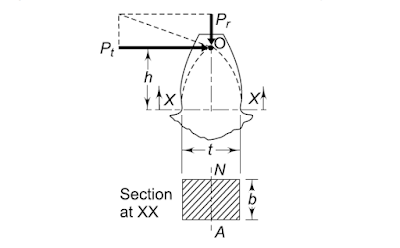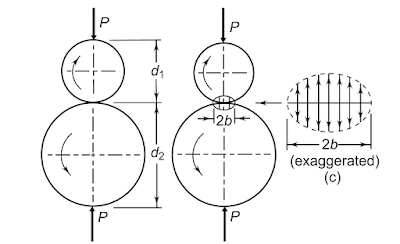# Design of Spur Gear, Material, Beam StrengthDesign of Spur Gear: Spur gears are used in machines, Design of gear for any machine is essential. Gear is defined as toothed wheels or multilobed cams.
Gear used to transmit power and motion from one shaft to another shaft by means of successive engagement of teeth.
Today we will discuss the Design of spur gear. Design means to develop a satisfactory solution for a given problem.
Let’s see why we need to design gear? There are lots of gears in every automatic or conventional machine; they work based on transfer motion and power from one component to another component.
Automatic machines have a high degree of accuracy in the range of some micron, Gears are used in the mechanism to smooth the operation. Thus it must be designed well.

## Design of Spur Gear according to Beam strength (Lewis equation)

Before I start, let's see the force acting at gearing action.

### Force analysis in Spur GearFrom the above figure, here Pt is tangential force, Pr is the radial force, and Pn is a normal force to the tooth gear.
Pt=Pncosa and Prsina
Pr=Pttana
Gear drive is generally specified by
·       Power to be transmitted
·       Speed of driving shaft
·       Velocity ratio
Power is transmitted by means of force exerted by the tooth of the driving gear on the matting teeth of the driven gear.
According to the law of gearing, this exerted force Pn is always normal to the tooth surface and acts along the pressure angle line.
Power P=Pt*V and Torque T=Pt*(D/2)

### Number of teeth

In the Design of spur gear it is require to decide number of teeth on pinion and gear.
Minimum number of teeth to avoid interference is given by
Zmin=2/sin2a
Once the number of teeth is decided on pinion, the number of teeth on gear can be calculated from the velocity ratio.In Lewis equation Gear the tooth is treated as a cantilever beam as shown in the figure.
The tangential component causes a bending moment about the base of the tooth.

### Lewis equation is based on the following assumptions

• Bending stress due to the radial component is negligible.
• Tangential component is uniformly distributed over the face of the width of gear.
• Stress concentration is negligible.
• Only one pair of teeth in contact.

Sigmab=(MY/I) , P=mbSigmabY
Y=Lewis form factor
Y=(t2/6hm)
The beam strength is the maximum value of tangential force that the tooth can transmit without bending.
Sb=mbSigmabY
In the Design of spur gear, it is require to decide weaker between pinon and gear.
Is is observed that the module and face width are same for the pinion and gear.
When same material used for the pinion and gear, the pinion is always weaker than gear.

## Design of Spur Gear as per Wear StrengthFailure of the gear tooth due to pitting occurs when contact stress between two meshing exceeds the surface endurance limits of the material.
The analysis of wear strength was done by Buckingham.
The equation is below
SigmaC=Maximum value of compressive strength.
P=force pressing the cylinder.
b=half width of deformation.
l=axial length of the cylinder.
d1,d2= diameters of cylinders.
E1,E2= Modulus of Elasticity